# Lightspeed Template Bootcamp Series (Lesson 3)

Moderator, Lightspeed Staff Posts: 654 moderator
edited May 2018
Lesson 3 - Javascript (JS)

Javascript is a programming language. It can affect the web in truly a dynamic way. Much like a machine, every part has its purpose in the whole.

First we’ll explore variables.

Variables are a way to store information. This include numbers

Below we will store some variables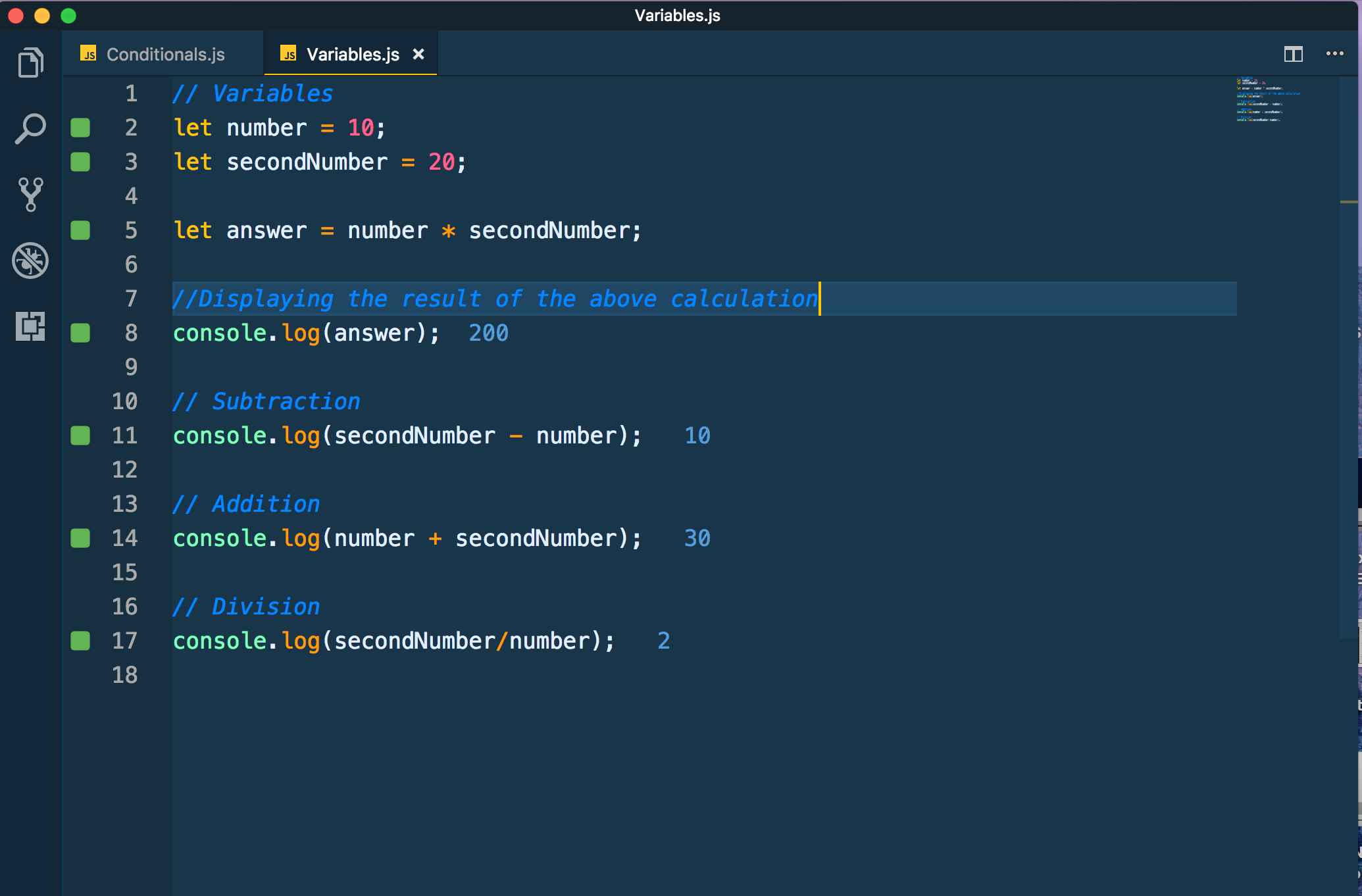In the above:

1. We created a variable called number and assigned it a value of 10
2. We created a variable called secondNumber and assigned it a value of 20
3. We multiplied the variables “number” and secondNumber together and stored it into a variable called “answer”
4. We displayed the result of the variable “answer”
5. We then did a number of mathematical operations on the variables

Below shows us writing conditional logic with Javascript: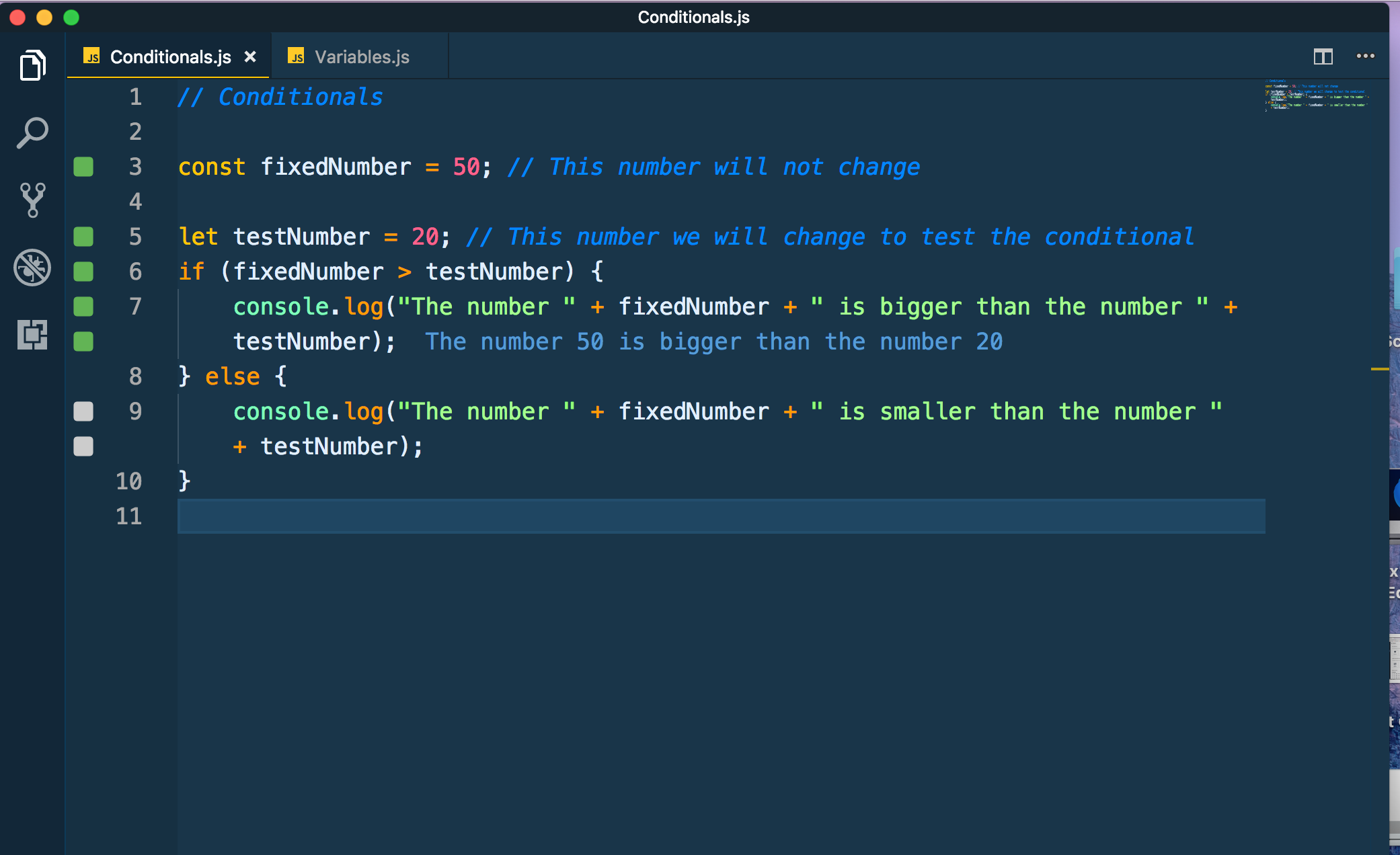The above explained:

1. First we assign a variable using the variable format const indicating that it is not going to change. fixedNumber is assigned a value of 50
2. Then we assign another variable called testNumber with the number 20
3. We then write an if condition: If the assigned value contained in the fixedNumber variable is greater than the assigned value of testNumber then print out a sentence, otherwise (else) if
4. You can see that the first condition is true since it printed out the statement “The number 50 is bugger than the number 20” in blue.

If I were to change the value assigned to the variable testNumber to something larger than the variable fixedNumber you would see how the conditional output changes: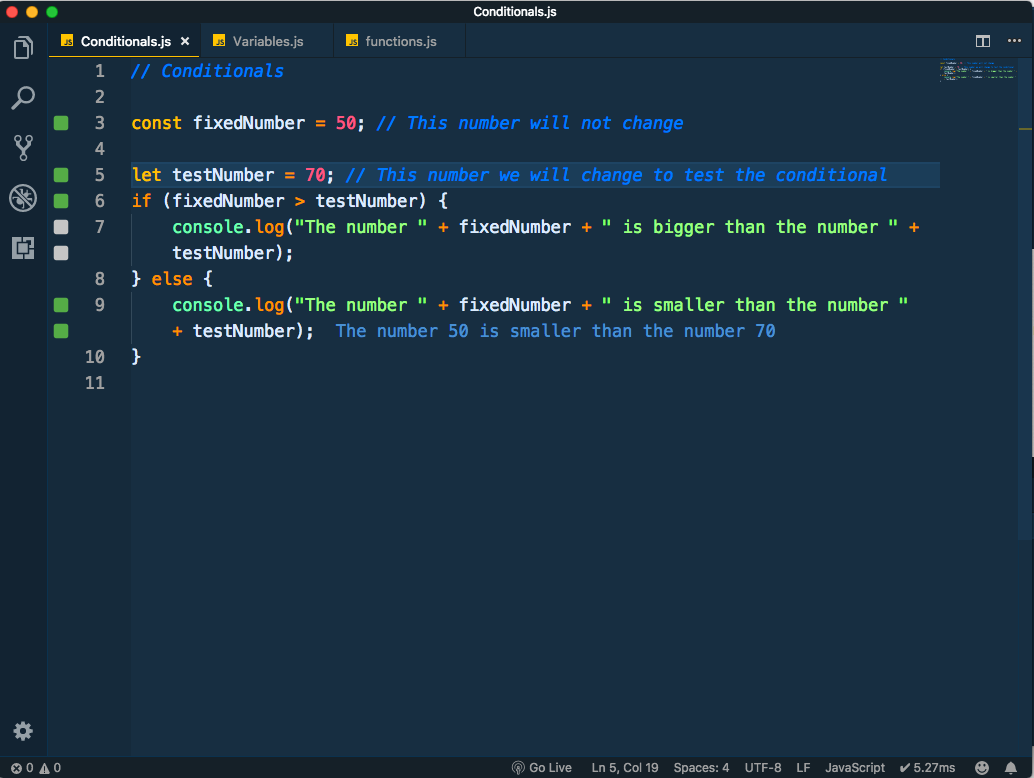Next we have functions. These are what I call micro-machines. They contain logic which can accept parameters. When the function is used, then it executes the function. We can create a function and use it as many times as we like in our code: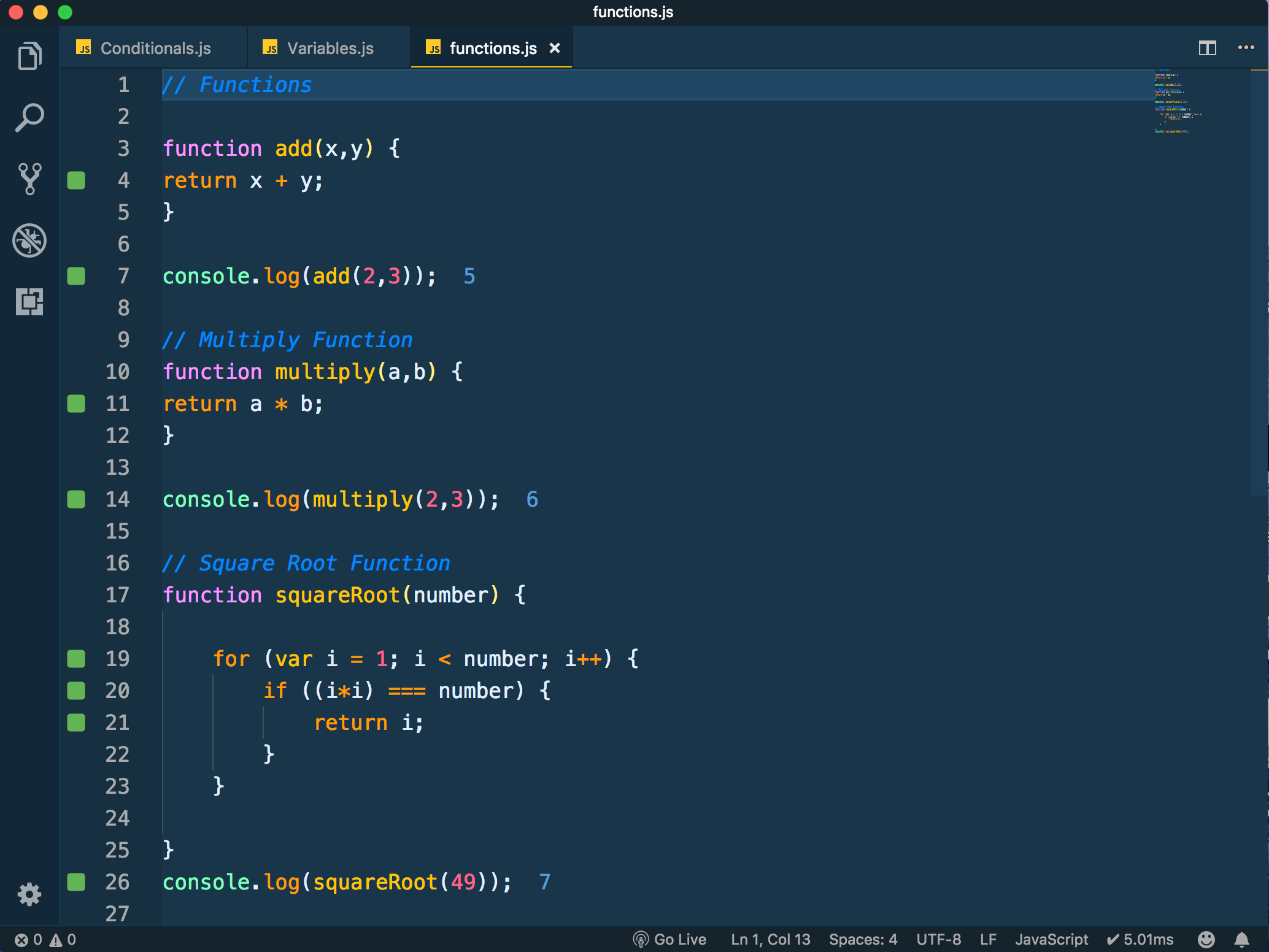1. First we created a function called “add” with two parameters x and y. Those two parameters were added together within the function.
2. We then use the function with real numbers and we console.log the output to see the result
3. Second we created a function called “multiply”, which accepts two parameters a and b. Those two parameters were multiplied together within a function
4. We then used the function with real numbers and we console.logged the output to see the result

5. The square root function is a bit more complicated. We only have one parameter, i.e. the number we want to find the square root of.
6. We then use a loop, which enables us to execute a function i number of times. In this instance it will be executed from 2 up to one digit before the number in question (because the square root can’t be one or itself).
7. We then write a conditional where if the iteration (i) squared (multiplied by itself) equals the number then we know this is the square root.
8. We then console.log the function to check whether our logic is correct.

Now we’ve explored some basics, let’s look at putting this all together:

I have the opportunity to execute scripts in the javascript console which is available on the web developer tools
You can navigate to this here: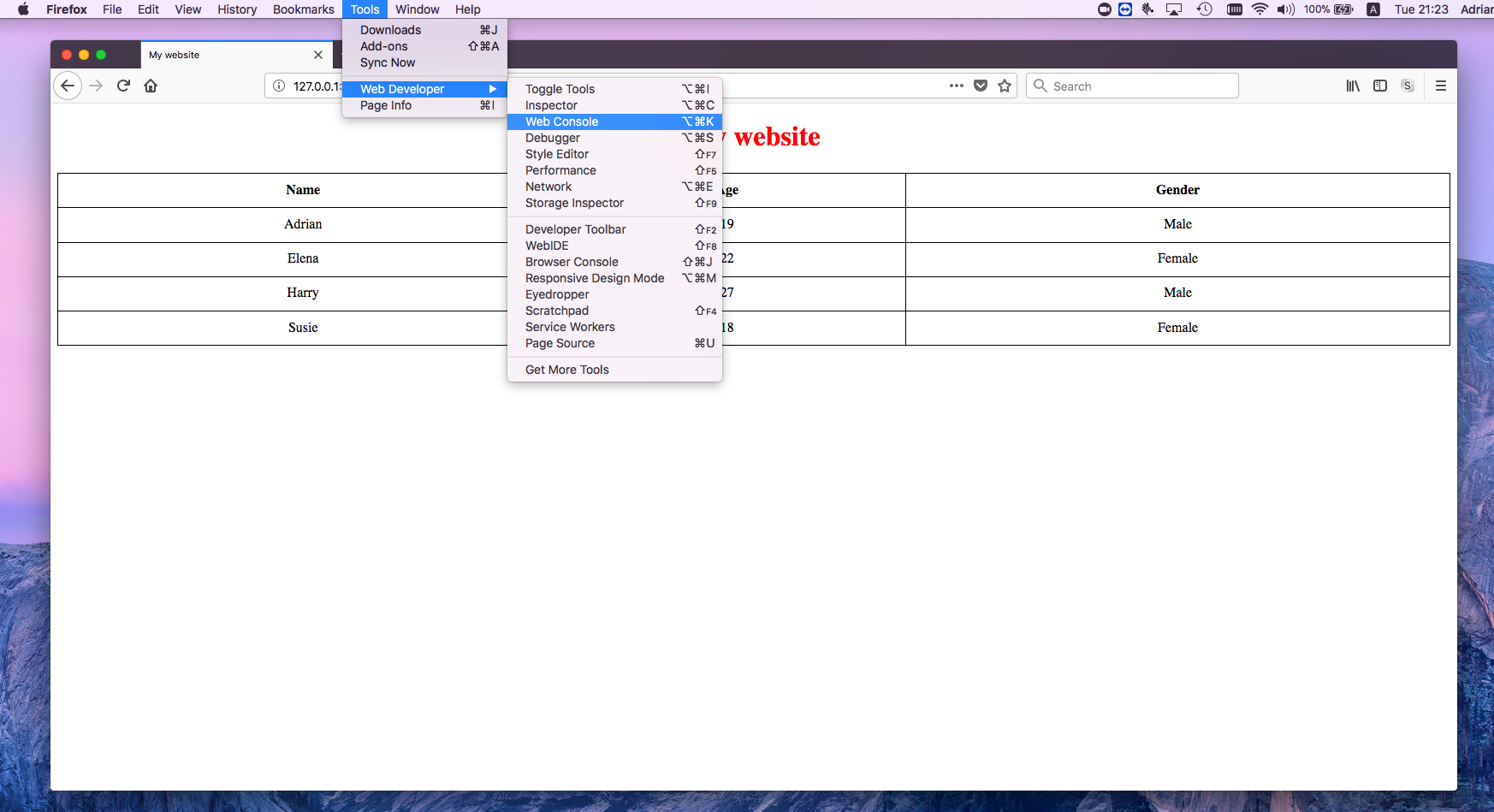We stored all the table tags in a variable and then logged the output. We can see the output of every td tag from the document. This is a useful way to test and experiment things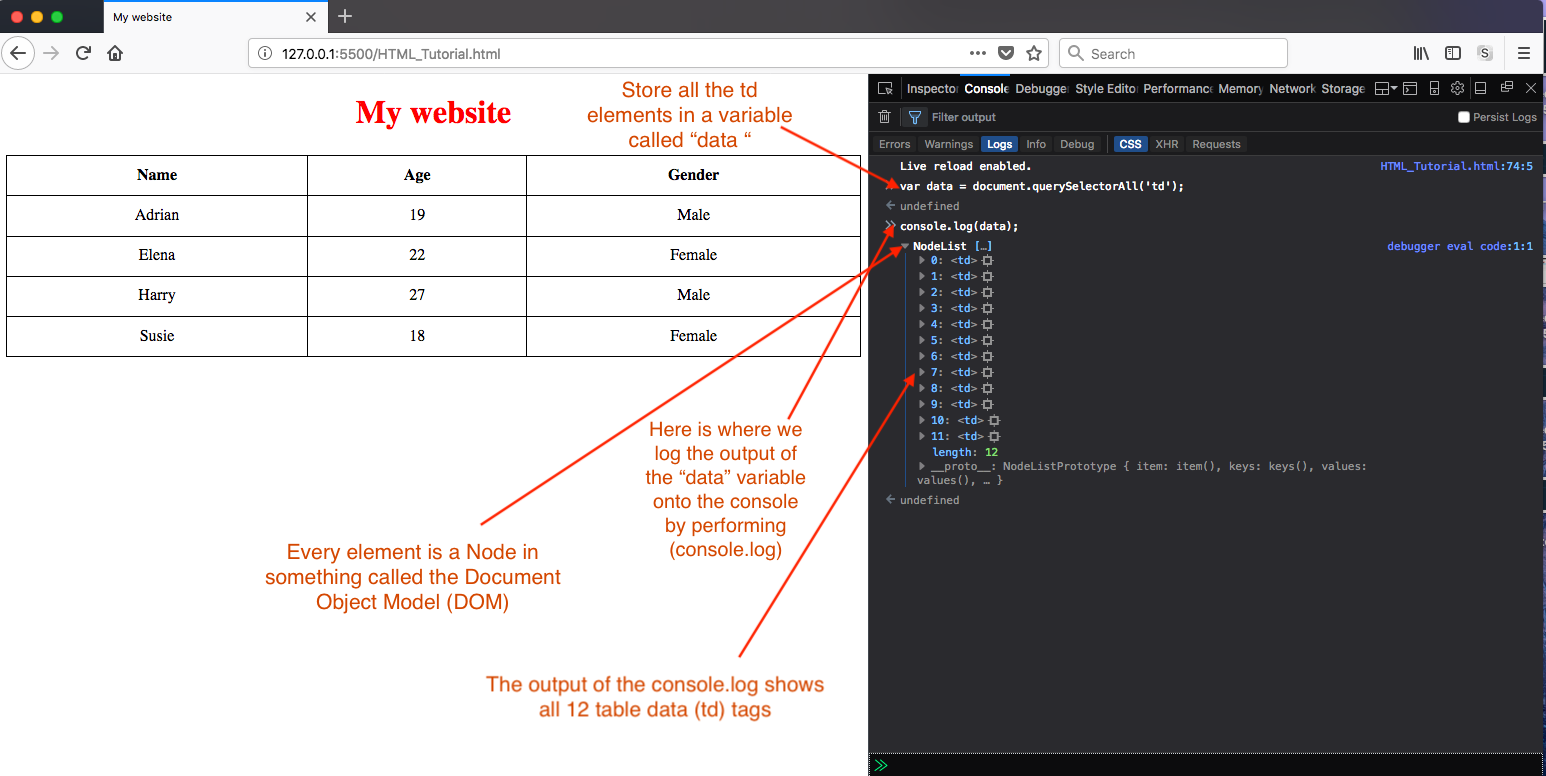Having decided on what I wanted to do, I craft a script. The code can be seen on the left, the results can be seen on the right.

Below contains a custom script combining these ideas together: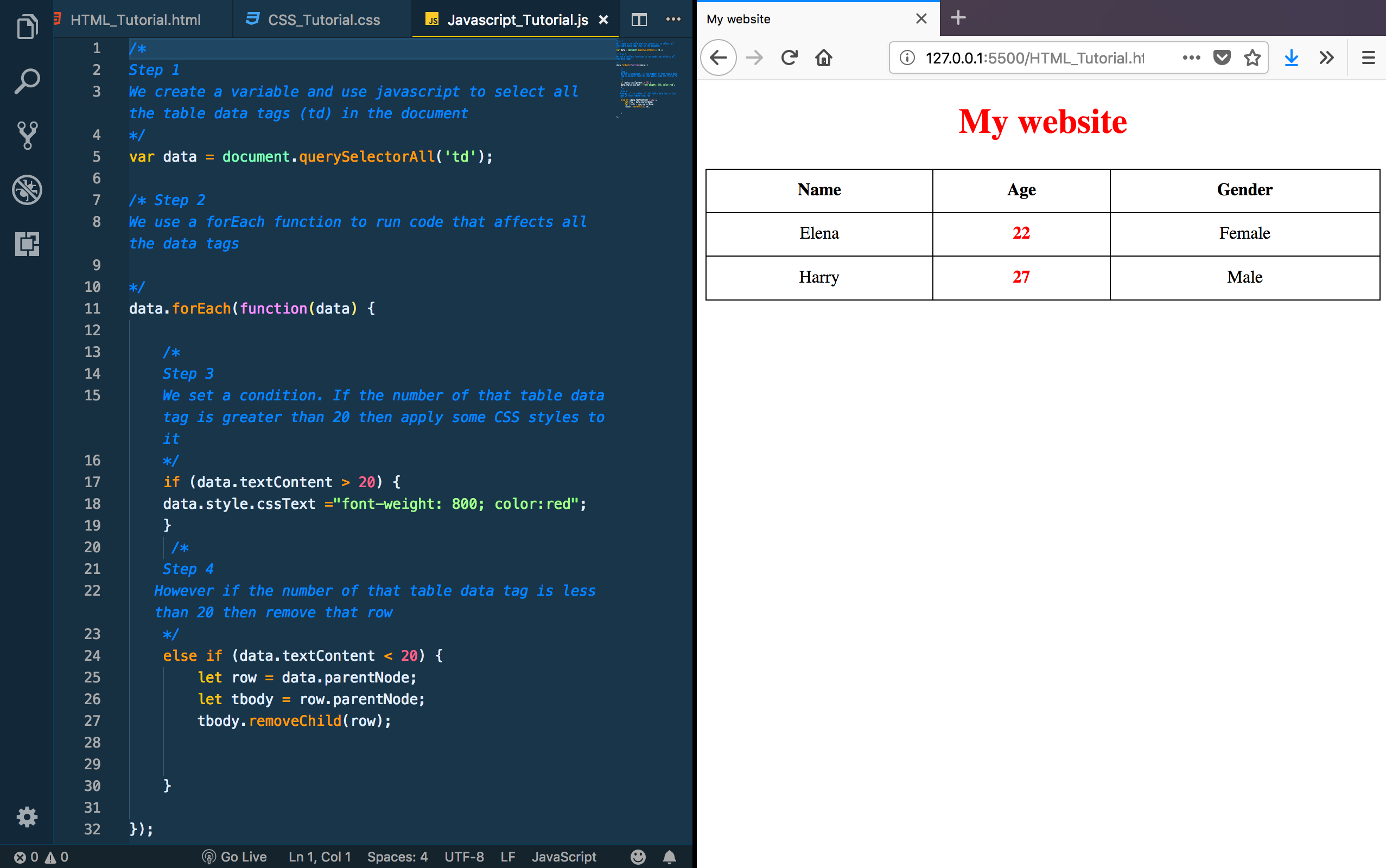Simply all this code does is test to see whether the data tags in the Age column are larger than 20 and apply CSS styling if it is or remove the column if it isn’t.

In detail:

1. We store all the table data tags in a variable called data.
2. We then operate a function that checks through every table data tag in the document
3. If the information inside the table data tag has a number that is greater than 20 then we add CSS to make the text bold and the colour red
4. However if the number inside the table data tag has a number less than twenty then we we store that row in a variable called row. We then store the parent element in a template called tbody. Then we remove the child of tbody (which is the row), thus the rows disappear that don’t fit the condition.

This at first might not seem that useful, but imagine data is being loaded into a table from a database. You are tasked to simply display only the information from people with an age greater than 20. This script would do just that. There are more practical use cases for our Lightspeed templates where we can dynamically change and amend data to suit or needs.

You can learn more about scripting with javascript from Mozilla’s documentation here: https://developer.mozilla.org/en-US/docs/Web/JavaScript

Next Lesson

Previous Lesson

Post edited by Adrian Samuel on

Adrian Samuel

Software Developer

Lightspeed HQ

Sign In or Register to comment.